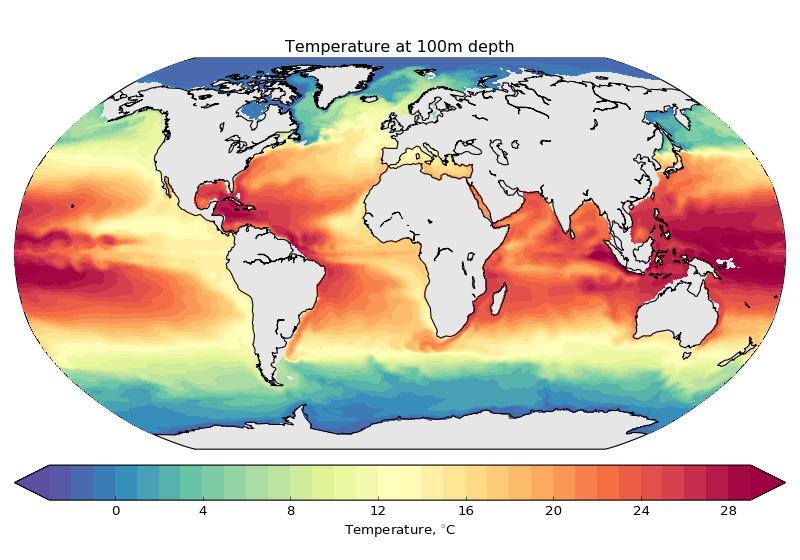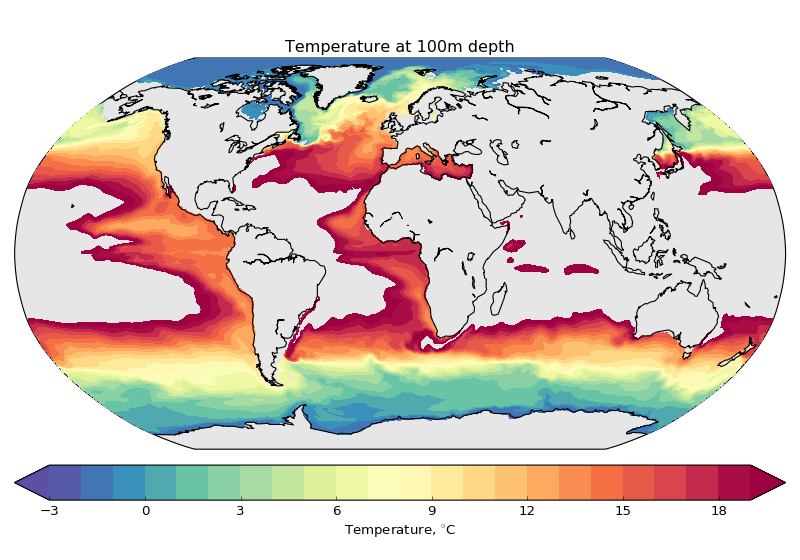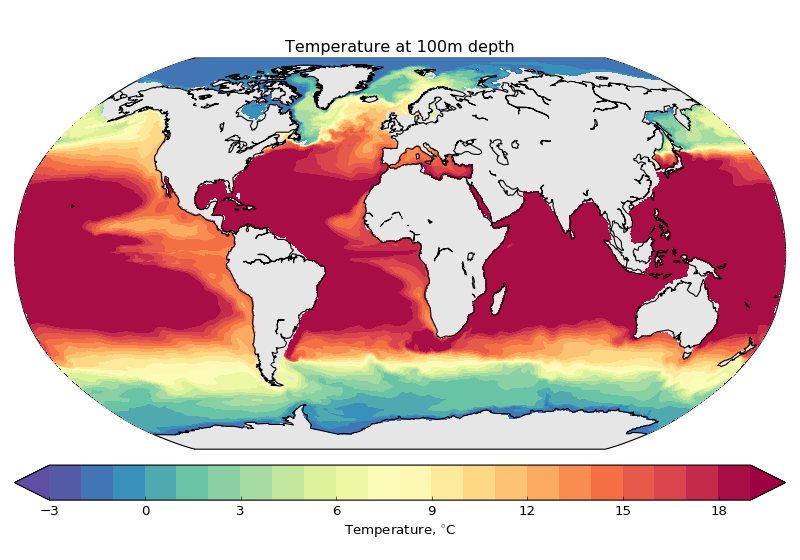# Plot variable on original FESOM grid¶

In many cases it is fine to first interpolate FESOM data to the regular grid and then plot them. Usually it will allow you to make plots faster. However sometimes you would like to work with original FESOM triangular mesh.

If you don't need interactivity on the plots, comment:

#%matplotlib notebook
In :
import sys
sys.path.append("../")

import pyfesom as pf
import matplotlib.pyplot as plt
from mpl_toolkits.basemap import Basemap
from matplotlib.colors import LinearSegmentedColormap
import numpy as np
%matplotlib notebook
from matplotlib import cm
from netCDF4 import Dataset


In :
meshpath  ='../../../../FESOM/mesh/'

The *usepickle = True* and the pickle file (*pickle_mesh*) exists.
We load the mesh from it.


In :
fl = Dataset('../../../../FESOM/data/fesom.2007.oce.nc')


There are several variables that we can plot:

In :
fl.variables.keys()

Out:
[u'time', u'iter', u'ssh', u'u', u'v', u'w', u'wpot', u'temp', u'salt']

For every timestep there is a vector of values, each coresponding to the node of the model mesh.

In :
fl.variables['temp'].shape

Out:
(12, 3668773)

In order to get values at some depth one should use get_data function from pyfesom. The input variables for this function are:

data : array
complete 3d data for one timestep
mesh : fesom_mesh object
mesh representation
depth : int
desired depth



As an output you will get:

level_data : array
2d array (actually vector) with data from the desired level.
elem_no_nan : array
array with triangles (defined as triplets of node indexes) with
not NaN elements.



The actual model level will be printed out.

Here is the result of requesting data from the level that is closest to 500 meters:

In :
level_data, elem_no_nan = pf.get_data(fl.variables['temp'][0,:], mesh, 500)

For depth 500 model level 490.0 will be used


The information from level_data and elem_no_nan is enought to make the plot:

Define the map:

In :
map = Basemap(projection='robin',lon_0=0, resolution='c')
x, y = map(mesh.x2, mesh.y2)


Plot the data from the last timestep of our dataset at 100m depth:

In :
%%time
level_data, elem_no_nan = pf.get_data(fl.variables['temp'][-1,:],mesh,100)

plt.figure(figsize=(10,7))
map.drawmapboundary(fill_color='0.9')
map.drawcoastlines()

levels = np.arange(-3., 30., 1)
plt.tricontourf(x, y, elem_no_nan[::], level_data, levels = levels, cmap=cm.Spectral_r, extend='both')
cbar.set_label("Temperature, $^{\circ}$C")
plt.title('Temperature at 100m depth')
plt.tight_layout()

For depth 100 model level 100.0 will be usedCPU times: user 1.3 s, sys: 28 ms, total: 1.33 s
Wall time: 1.45 s


If your color range covers the whole range of your data everything is fine. But if you try to narrow the range, the places with values outside your range become masked out:

In :
%%time
level_data, elem_no_nan = pf.get_data(fl.variables['temp'][-1,:],mesh,100)

plt.figure(figsize=(10,7))
map.drawmapboundary(fill_color='0.9')
map.drawcoastlines()

levels = np.arange(-3., 20., 1)
plt.tricontourf(x, y, elem_no_nan[::], level_data, levels = levels, cmap=cm.Spectral_r, extend='both')
cbar.set_label("Temperature, $^{\circ}$C")
plt.title('Temperature at 100m depth')
plt.tight_layout()

For depth 100 model level 100.0 will be usedCPU times: user 820 ms, sys: 20 ms, total: 840 ms
Wall time: 841 ms


The way around it is to chnage your data :)

In :
%%time
level_data, elem_no_nan = pf.get_data(fl.variables['temp'][-1,:],mesh,100)

plt.figure(figsize=(10,7))
map.drawmapboundary(fill_color='0.9')
map.drawcoastlines()

levels = np.arange(-3., 20., 1)

eps=(levels.max()-levels.min())/50.
level_data[level_data<=levels.min()]=levels.min()+eps
level_data[level_data>=levels.max()]=levels.max()-eps
plt.tricontourf(x, y, elem_no_nan[::], level_data, levels = levels, cmap=cm.Spectral_r, extend='both')
cbar.set_label("Temperature, $^{\circ}$C")
plt.title('Temperature at 100m depth')
plt.tight_layout()

For depth 100 model level 100.0 will be used/home/magik/miniconda2/lib/python2.7/site-packages/ipykernel/__main__.py:10: RuntimeWarning: invalid value encountered in less_equal
/home/magik/miniconda2/lib/python2.7/site-packages/ipykernel/__main__.py:11: RuntimeWarning: invalid value encountered in greater_equal

CPU times: user 824 ms, sys: 72 ms, total: 896 ms
Wall time: 896 ms


The things above were easy - since it was just doing contour plots. But sometimes it is nessesary to have a look at the data on original grid. Since properties in FESOM 1 are defined on the verticies of the triangles, the values for making the colors of the triangles will be calculated as a mean of the values in the verticies.

In :
%%time
level_data, elem_no_nan = pf.get_data(fl.variables['temp'][-1,:],mesh,100)

plt.figure(figsize=(10,7))
map.drawmapboundary(fill_color='0.9')
map.drawcoastlines()

plt.tripcolor(x, y, elem_no_nan, \
level_data, \
edgecolors='k',\
lw = 0.01,
cmap=cm.Spectral_r,
vmin = -3,
vmax = 30)
cbar.set_label("Temperature, $^{\circ}$C")

For depth 100 model level 100.0 will be used